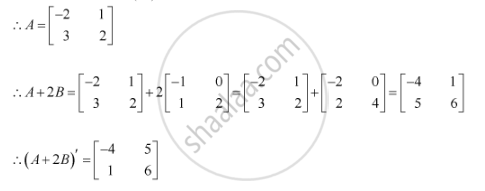Share

# If A' =Matric [(-2,3),(1,2)] and B = Matric [(-1,0),(1,2)] Then Find (A + 2b)' - CBSE (Commerce) Class 12 - Mathematics

ConceptSymmetric and Skew Symmetric Matrices

#### Question

if A' = [(-2,3),(1,2)] and B = [(-1,0),(1,2)]  then find (A + 2B)'

#### Solution

We know that A = (A')'Is there an error in this question or solution?

#### Video TutorialsVIEW ALL 

Solution If A' =Matric [(-2,3),(1,2)] and B = Matric [(-1,0),(1,2)] Then Find (A + 2b)' Concept: Symmetric and Skew Symmetric Matrices.
S